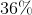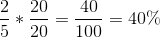## Example Questions

### Example Question #1 : Fractions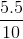is equivalent to what percentage?

55%

45%

15%

4.5%

5.5%

55%

Explanation:

Divide the fraction out to get the decimal

5.5 / 10 = 0.55

To find the percentage, shift the decimal point two to the right = 55%

### Example Question #2 : Fractions

Write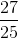as a percent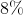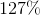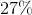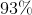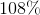Explanation:

To find a percent from a fraction you can either divide the number on your calculator and multiply by 100: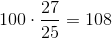or you can just multiply the numerator and denominator by the same number that makes the denominator equal to 100 (because a % is just #/100):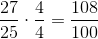### Example Question #1 : How To Find Percentage From A Fraction A1

One half of a group of professors is made up of mathematicians, and one fourth of the remaining portion is made up of philosophers. The remaining portion is made up of sociologists. If there are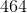professors in the room, how many are sociologists?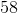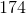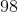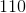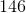Explanation:

To begin, remember with percentages that "of" means multiplication and "is" means "equals." Now, we know that one fourth of the remaining half are philosophers. This means that he philosophers really are: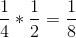So, there are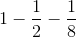sociologists as a percentage. This is: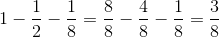Therefore,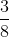of theare sociologists, or: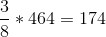are sociologists.

### Example Question #4 : Fractions

Convert the following fraction into a percent.